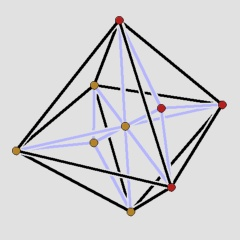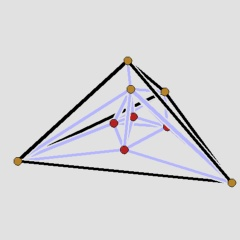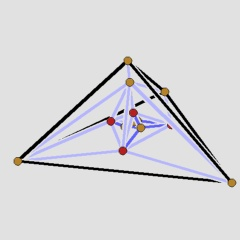Smallest non-trivial 2s2s-polytopeElectronic Geometry Model No. 2005.12.001

Axel Werner

Description

The smallest non-trivial 2-simplicial and 2-simple 4-polytope.

A polytope P is k-simplicial if all its k-faces are simplices and it is h-simple if its dual polytope PΔ is h-simplicial . Therefore, a 4-dimensional polytope is 2-simplicial and 2-simple, if all 2-dimensional faces are triangles and all edges are contained in exactly 3 facets; in this case, we call it a 2s2s-polytope. Trivially, the 4-simplex is a 2s2s-polytope.

The flag vectors of 2s2s-polytopes satisfy two linear inequalities, valid for all flag vectors of 4-polytopes, with equality and hence are contained in a 2-dimensional face of the flag vector cone of 4-polytopes . It was first shown by Paffenholz and Ziegler  that there exist infinitely many 2s2s-polytopes on an arbitrarily large number of vertices.

It is then natural to ask for the existence of 2s2s-polytopes on few vertices. The polytope W9 on 9 vertices, of which a Schlegel diagram is given in the first figure, is the combinatorially unique smallest non-trivial 2s2s-polytope.

This can be proved by elementary methods in several steps. Let P be a 2s2s-polytope with at most 9 vertices that is not the simplex; then one can show:

• P cannot have less than 9 vertices.
• P contains at most one facet with 6 vertices.
• if P contains an octahedron then it is combinatorially equivalent to W9.
• P or PΔ contains an octahedron.
• W9 is self-dual.
These assertions together imply the claim.

The polytope given here generalises to a whole family of 2s2s-polytopes with a further, very interesting property. A d-polytope is elementary if it satisfies g2=0, where

g2 = f02 - 3 f2 + f1 - d f0 + d(d+1)/2

with the (reduced) flag vector (f0,f1,f2;f02). Kalai  showed that g2>=0 is a valid linear inequality for all flag vectors of 4-polytopes. Elementary 2s2s-polytopes can therefore be found on a ray l1 of the flag vector cone (see ).

Until recently, only two flag vectors of polytopes were known to lie on l1. The polytope W9 is elementary, which can be easily calculated from its f-vector (9,26,26,9) and from the fact that it is 2-simplicial (i.e. f02=3f2). By performing a sequence of "pseudo-stacking steps", that is, placing new vertices beyond certain facets in a suitable way, one can construct elementary 2s2s-polytopes W4k+1 on 4k+1 vertices for all k>=1. The construction is described in detail in , together with other examples that cover all vertex numbers n>=9, n≠12.

The second figure shows a different Schlegel diagram of W9. Pseudo-stacking starts beyond the simplex facet, whose vertices are coloured red in all the figures, and adds four vertices. This yields the next example, W13, depicted in the third figure, with new edges arising in the pseudo-stacking process hilighted.

 Keywords Polytope; Discrete Geometry MSC-2000 Classification 52B05 Zentralblatt No. 05264906

References

1. Bayer, Margaret M.: The Extended f-Vectors of 4-Polytopes, J. Combin. Theory Ser. A 44 (1987), 141--151.
2. Grünbaum, Branko: Convex Polytopes, 2nd edition, Prepared and with a preface by Volker Kaibel, Victor Klee and Günter M. Ziegler, Springer-Verlag (2003).
3. Kalai, Gil: Rigidity and the lower bound theorem. I, Invent. Math. 88 (1987), 125--151.
4. Paffenholz, Andreas and Werner, Axel: Constructions for 4-Polytopes and the Cone of Flag Vectors, Cont. Math. 423 (2007), 283--303, http://de.arxiv.org/abs/math.CO/0511751.
5. Paffenholz, Andreas and Ziegler, Günter M.: The E-construction for lattices, spheres and polytopes, Discrete Comput. Geom. 32 (2004), 601--621.

Submission information

Submitted: Wed Dec 14 15:51:58 CET 2005.
Revised: Mon Mar 19 16:51:00 CET 2007.
Accepted: Tue Jun 5 12:50:52 CEST 2007.